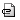Up:Tutorial?　　 　Previous：Wheeled mobile robot　　　 Next: Eye movement

## Distance sensor †

This tutorial is valid for version later than v2.1.0.

Distance sensor uses camera (depth image). This page explains how to get distance information from SIGViewer service.

There are three types of distance information: 1) distance from the camera and a target object (scalar) 2) distance array measured along a horizontal plane (vector) 3) depth map (2D matrix)

### 1) scalar distance †

This sample uses a function distanceSensor() to get a distance between the camera and a target object which is gazed by the camera.

#### Controller †

Move to the working directory and edit a controller.

```\$ cd ~/MyWorld
\$ emacs distanceSensor.cpp```

distanceSensor.cpp

The distanceSensor() in the 62nd line gets the distance.

The type of the return value is unsigned char, that indicates from 0 to 255. Maximum distance is 255cm. If a true distance is longer than 255cm, the return value will be 255. The maximum distance can be changed by argument of distanceSensor() as shown below:

change

to

The first and second argument indicate range of the distance sensor. The first argument is offset value. The second argument is range of measurable area. In the above example case, the distance sensor can measure from 50cm to 550cm. The range of the return value is still from 0 to 255. If the distance is shorter than 50cm, the return value will be 0; if the distance is longer than 550cm, the return value will be 255. Resolution of the distance sensor will be rough, if you choose wide range.

The last argument is ID number of camera. If you omit this argument, the distance sensor will be corresponded to the camera which ID is No.1.

In this controller, a robot keeps on going forward; if the distance is shorter than 100cm, the direction will be changed randomly.

Compile the source code of the controller.

`\$ ./sigmake.sh distanceSensor.cpp`

#### World File †

Next, edit a world file

`\$ emacs distanceSensor.xml`

distanceSensor.xml

This time, a wheeled mobile robot is used.

#### Execution †

Execute with the following command

`\$ sigserver.sh -w ./distanceSensor.xml`

The robot will go forward; change the direction in front of obstacles.

### Measurement of distance vector †

Next, an example that measure distance vector along a horizontal plane.

#### Modification of Controller †

Edit another new controller

`\$ emacs distanceSensor1D.cpp`

distanceSensor1D.cpp

In this example, the robot changes direction when the shortest distance in the distance vector is shorter than 100cm.

distanceSensor1D() gets the distance vector along a horizontal plane which position is identical to a target camera. Class of the return value is ViewImage.

The range of the measurable angle is identical to the horizontal angle of camera view. The size of the vector is 320 in initial setting; each array element is corresponded to each pixel. getBuffer() should be used to get each distance value from the array. array corresponds to the leftmost pixel; array corresponds to the rightmost pixel.

#### Seeting of view angle †

You can change the horizontal view angle with vertical view angle and aspect ratio in a world file.

Due to the resolution quality, the recommended horizontal angle is from 30(deg) to 80(deg).

#### Compile †

Enter the following command to compile.

`\$ ./sigmake.sh distanceSensor1D.cpp`

#### Edit of the world file †

Change the controller to distanceSensor1D.so

`\$ emacs distanceSensor.xml`

↓

#### Execution †

Enter the following command to execute.

`\$ sigserver.sh  -w ./distanceSensor.xml`

In this example, quality of the avoidance behavior becomes better. The robot cannot escape from the four obstacles.

### Measurement of 2D matrix distance †

This section explains how to get the 2D matrix distance.

#### Edit of controller †

Edit another new controller.

`\$ emacs distanceSensor2D.cpp`

distanceSensor2D.cpp

distanceSensor2D() gets the distance matrix.

In this sample, theta (vertical angle) and phi (horizontal angle) in polar coordinate are calculated from pixel position.

Use the following function to save the depth map image as well as captureView().

#### Edit of world file †

`\$ emacs distanceSensor.xml`

Change the controller from distanceSensor1D.so to distanceSensor2D.so.

#### Execution †

Enter the following command to execute.

`\$ sigserver.sh -w ./distanceSensor.xml`

The depth map image will be created as shown below:

Further distance is shown by dark; shorter distance is shown by light.

Up:Tutorial?　　 　Previous：Wheeled mobile robot　　　 Next: Eye movement

Counter: 4377, today: 1, yesterday: 0

Attach file:distance.jpg 1561 downloaddistance_1.PNG 1811 download

Last-modified: 2014-02-07 (Fri) 00:54:14 (3025d)